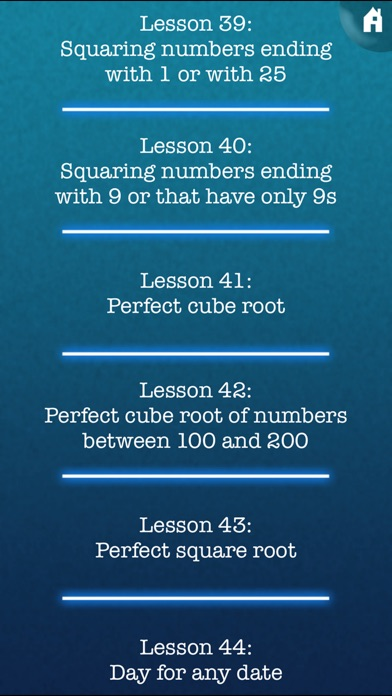# Mimir Mental Math

## iOS UniversalEducation

\$1.99

Would you like to improve your mental math skills? Would you like to learn how to solve difficult math problems with ease and impress yourself and your friends with your math skills? With this app you will not only train your brain by solving math problems, you will also learn special mental math tricks.

For many math problems, special tricks exist that make solving these much easier. It is important to know when to use which trick, but also how to solve a math problem if none of these special tricks can be used.

This app contains 44 lessons (with up to 3 tricks per lesson) where you will learn tips and tricks for the following types of math problems:
-Subtraction
-Multiplication
-Division
-Divisibility
-Remainders
-Squaring
-Square and cube roots
-Calculate the weekday for any date

You will not only learn the special tricks, but also more general solutions.

In the second part of this app, the training, you can solve random math problems with increasing difficulty (adaptive training). Or you can select what type of math problem you want to practice, and in which range the numbers should be (training with choice). The goal here is not only to become better and faster at solving math problems, you will also learn to detect which trick would be the best for the current math problem. This is why there is a help button in the training-section. If you push this button, the application will search for the trick or tip that is possibly the easiest for solving the current math problem.

-Two languages: All texts are in english and german.
-User management: Multiple users can use the same device. The application saves the settings and position in the training for each user separately.
-Two different GUI-designs: Use a blue flickering background with white text in sci-fi movie style. Or switch to a simple white paper background with black text.

Lessons:
Introduction
Subtraction
Single digit multiplications
x 10 and x 5
x 2, x 4 and x 8
x 9 and x 3
x 6 and x 7
x 11 and x 12
Multiplication of numbers between 11 - 19 and between 91 - 99
Multiplication of numbers close to a power of 10
Multiplication of numbers close to multiples of 100 or 1000
Multiplying numbers with two different bases
Multiplication of 2 digit numbers
Multiplication of 3 digit numbers
x 111, x 21 and x 121
x 101 and x 1001
x 15, x 25 and x 50
x 95 and x 125
x 2-digit numbers that end in 5 and x 50 to 59
x 99, x 999 and x 999999…
x 19 and x 2-digit numbers that end in 9 (including a special case)
÷ 10, ÷ 5 and ÷ 4
÷ 9 and ÷ 8
Division: Remainders method
General division method
Divisibility by 2, 5 and 10
Divisibility by 9, 3 and 6
Divisibility by 4, 8 and 7
Divisibility by 11, 12 and 13
Remainder when dividing by 2, 5 and 10
Remainder when dividing by 3, 9 and 6
Remainder when dividing by 4 and 8
Remainder when dividing by 7 and 11
Squaring 1 to 29
Squaring numbers ending with 5, and 50 to 59
Squaring 26 to 125
Squaring numbers close to 1000, and general squaring method
Squaring numbers ending with 1 or with 25
Squaring numbers ending with 9 or that have only 9s
Perfect cube root
Perfect cube root of numbers between 100 and 200
Perfect square root
Day for any date

## What's New

Better custom training options

•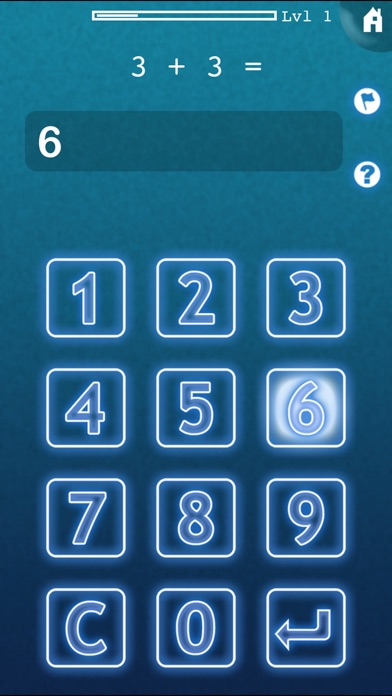•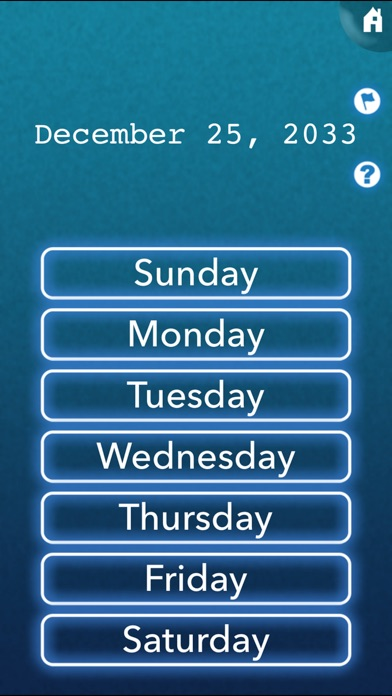•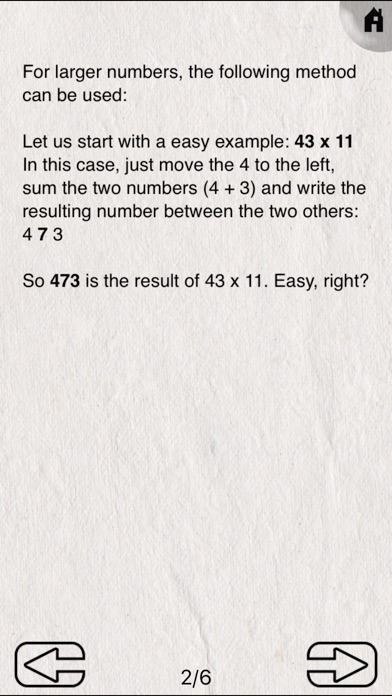•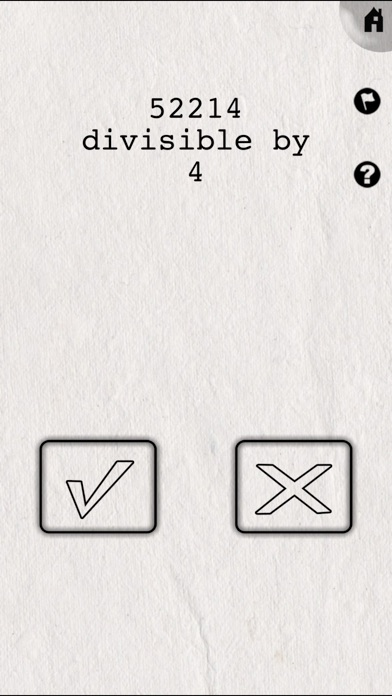•